Next: Polarization of Electromagnetic Waves Up: Multi-Dimensional Waves Previous: Spherical Waves

# Oscillation of an Elastic Sheet

A straightforward generalization of the analysis of Section 5.2 reveals that the transverse oscillation of a uniform elastic sheet, stretched over a rigid frame, is governed by the two-dimensional wave equation (Pain 1999)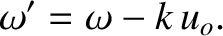(542)

Here,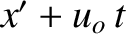is the sheet's transverse (i.e., in the-direction) displacement,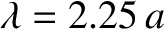the characteristic speed of elastic waves on the sheet,the tension, and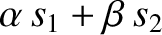the mass per unit area. In equilibrium, the sheet is assumed to lie in the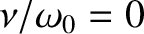-plane. The boundary condition is thatat the rigid frame.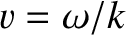Suppose that the frame is rectangular, extending from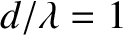to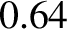, and from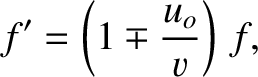to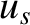. Let us search for a normal mode of the form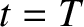(543)

Substitution into Equation (542) yields(544)

subject to the boundary conditions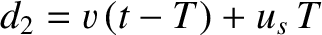. Let us search for a separable solution of the form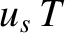(545)

Such a solution satisfies the boundary conditions provided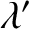. It follows that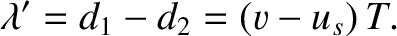(546)

The only way that the preceding equation can be satisfied at allandis if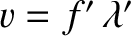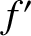(547)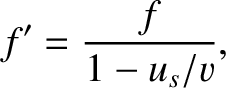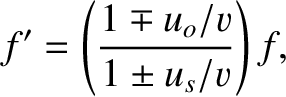(548)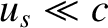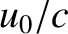(549)

where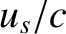and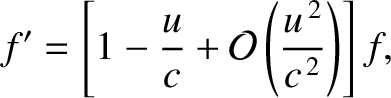are positive constants. (The constants have to be positive, rather than negative, to give oscillatory solutions that are capable of satisfying the boundary conditions.) Appropriate solutions of Equations (547) and (548) are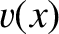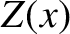(550)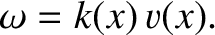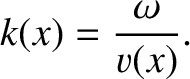(551)

where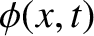and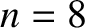are arbitrary constants. These solutions automatically satisfy the boundary conditions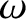. The boundary conditions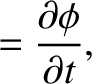are satisfied provided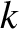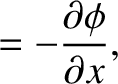(552)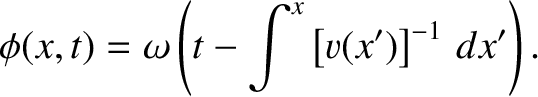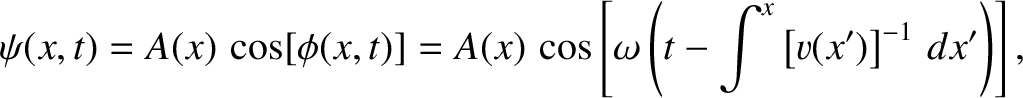(553)

where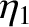and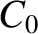are positive integers. Thus, the normal modes of a rectangular elastic sheet, which are indexed by the mode numbersand, take the form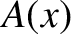(554)

where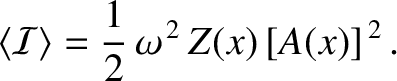(555)

Here,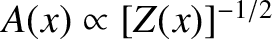and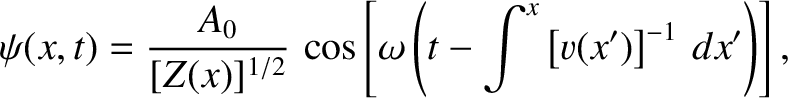are arbitrary constants. Because Equation (542) is linear, its solutions are superposable. Hence, the most general solution is a superposition of all of the normal modes: that is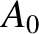(556)

The amplitudes, and the phase angles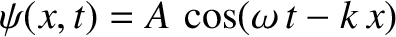, are determined by the initial conditions. (See Exercise 4.) Figure 41 illustrates the spatial variation of the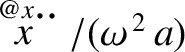,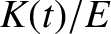normal mode of a rectangular elastic sheet with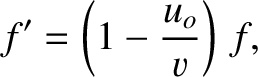.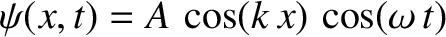Suppose that an elastic sheet is stretched over a circular frame of radius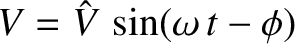. Defining the cylindrical coordinate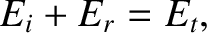, where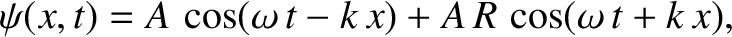corresponds to the location of the frame, the axisymmetric oscillations of the sheet are governed by the cylindrical wave equation (see Section 8.3)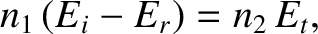(557)

subject to the boundary conditionat. Let us search for a normal mode of the form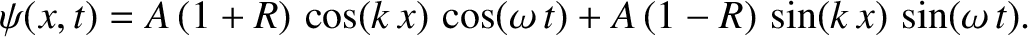(558)

Substitution into Equation (557) yields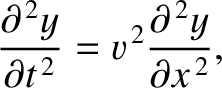(559)

subject to the boundary condition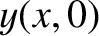. Let define the scaled radial coordinate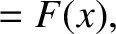. When written in terms of this new coordinate, the above equation transforms to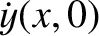(560)

This well-known equation is called Bessel's equation (of degree zero), and has the standard solution (that is well-behaved at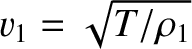) (Abramowitz and Stegun 1965)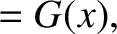(561)

Here,is termed a Bessel function (of degree zero), and is plotted in Figure 42. It can be seen that the function is broadly similar to a cosine function, except that its zeros are not quite evenly spaced, and its amplitude gradually decreases asincreases. The first few values ofat which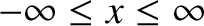are listed in Table 3. Let the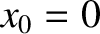th zero be located at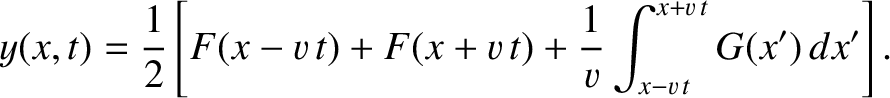. In order to satisfy the boundary condition, we require that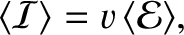. Hence, the axisymmetric normal modes of an elastic sheet, stretched over a circular frame of radius, are indexed by the mode number(which is a positive integer), and take the form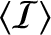(562)

where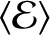(563)

and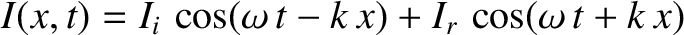,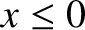are arbitrary constants. Figure 43 illustrates the spatial variation of the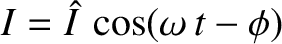normal mode.

Table: First few zeros of the Bessel function. Source: Abramowitz and Stegun 1965.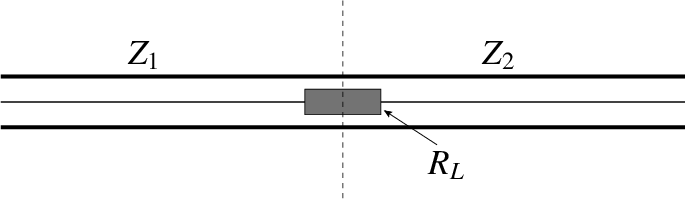1 2.40482 6 18.07106 2 5.52007 7 21.21163 3 8.65372 8 24.35247 4 11.79153 9 27.49347 5 14.93091 10 30.63460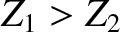Next: Polarization of Electromagnetic Waves Up: Multi-Dimensional Waves Previous: Spherical Waves
Richard Fitzpatrick 2013-04-08